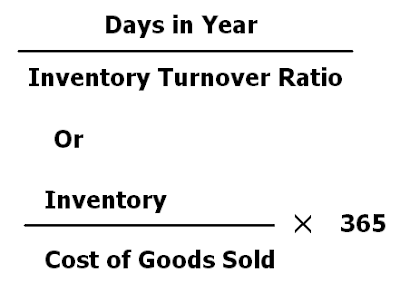Inventory conversion period reports us about the average time to convert our total inventory into sales. It is relationship between total days in year and inventory turnover ratio. In other words, it measures the length of time on average between the acquisition and sale of merchandise. We can calculate it with following formula.

For example, inventory turnover ratio is 10 times of average stock at cost. Its inventory conversion period will be

= 365/ 10 = 37 days. It means, the inventory has been disposed off or sold on an average in 37 days.

Interpretation of Inventory Conversion Period

1. Less inventory conversion period is better because more fastly, we will convert our inventory into sales, there will be less chance of obsolescence and paying of over-stocking cost.

2. Inventory conversion period is the part of cash conversion cycle. If this period is very high, it will increase the time to complete  the cash conversion cycle. It means, there will be more liquidity risk in that level of inventory.

3. After adding average collection period and deducting average payment period, we can take good decision relating to inventory level. Following example will explain its importance  in simple way..

## \$type=three\$a=hide\$cm=hide\$s=hide\$show=/2019/06/10-steps-to-become-better-investor.html\$l=hide

Name

false
ltr
item
Accounting Education: Inventory Conversion Period
Inventory Conversion Period
http://4.bp.blogspot.com/-8zjditg_F20/Tr0a5kIxAPI/AAAAAAAAHDA/gjpbhdBv6CU/s400/pics+inventory+turnover+ratio.PNG
http://4.bp.blogspot.com/-8zjditg_F20/Tr0a5kIxAPI/AAAAAAAAHDA/gjpbhdBv6CU/s72-c/pics+inventory+turnover+ratio.PNG
Accounting Education
http://www.svtuition.org/2011/11/inventory-conversion-period.html
http://www.svtuition.org/
http://www.svtuition.org/
http://www.svtuition.org/2011/11/inventory-conversion-period.html
true
2410664366776677676
UTF-8